Chemistry 2e

# Exercises

Chemistry 2eExercises

### 7.1Ionic Bonding

1.

Does a cation gain protons to form a positive charge or does it lose electrons?

2.

Iron(III) sulfate [Fe2(SO4)3] is composed of Fe3+ and $SO42−SO42−$ ions. Explain why a sample of iron(III) sulfate is uncharged.

3.

Which of the following atoms would be expected to form negative ions in binary ionic compounds and which would be expected to form positive ions: P, I, Mg, Cl, In, Cs, O, Pb, Co?

4.

Which of the following atoms would be expected to form negative ions in binary ionic compounds and which would be expected to form positive ions: Br, Ca, Na, N, F, Al, Sn, S, Cd?

5.

Predict the charge on the monatomic ions formed from the following atoms in binary ionic compounds:

(a) P

(b) Mg

(c) Al

(d) O

(e) Cl

(f) Cs

6.

Predict the charge on the monatomic ions formed from the following atoms in binary ionic compounds:

(a) I

(b) Sr

(c) K

(d) N

(e) S

(f) In

7.

Write the electron configuration for each of the following ions:

(a) As3–

(b) I

(c) Be2+

(d) Cd2+

(e) O2–

(f) Ga3+

(g) Li+

(h) N3–

(i) Sn2+

(j) Co2+

(k) Fe2+

(l) As3+

8.

Write the electron configuration for the monatomic ions formed from the following elements (which form the greatest concentration of monatomic ions in seawater):

(a) Cl

(b) Na

(c) Mg

(d) Ca

(e) K

(f) Br

(g) Sr

(h) F

9.

Write out the full electron configuration for each of the following atoms and for the monatomic ion found in binary ionic compounds containing the element:

(a) Al

(b) Br

(c) Sr

(d) Li

(e) As

(f) S

10.

From the labels of several commercial products, prepare a list of six ionic compounds in the products. For each compound, write the formula. (You may need to look up some formulas in a suitable reference.)

### 7.2Covalent Bonding

11.

Why is it incorrect to speak of a molecule of solid NaCl?

12.

What information can you use to predict whether a bond between two atoms is covalent or ionic?

13.

Predict which of the following compounds are ionic and which are covalent, based on the location of their constituent atoms in the periodic table:

(a) Cl2CO

(b) MnO

(c) NCl3

(d) CoBr2

(e) K2S

(f) CO

(g) CaF2

(h) HI

(i) CaO

(j) IBr

(k) CO2

14.

Explain the difference between a nonpolar covalent bond, a polar covalent bond, and an ionic bond.

15.

From its position in the periodic table, determine which atom in each pair is more electronegative:

(a) Br or Cl

(b) N or O

(c) S or O

(d) P or S

(e) Si or N

(f) Ba or P

(g) N or K

16.

From its position in the periodic table, determine which atom in each pair is more electronegative:

(a) N or P

(b) N or Ge

(c) S or F

(d) Cl or S

(e) H or C

(f) Se or P

(g) C or Si

17.

From their positions in the periodic table, arrange the atoms in each of the following series in order of increasing electronegativity:

(a) C, F, H, N, O

(b) Br, Cl, F, H, I

(c) F, H, O, P, S

(d) Al, H, Na, O, P

(e) Ba, H, N, O, As

18.

From their positions in the periodic table, arrange the atoms in each of the following series in order of increasing electronegativity:

(a) As, H, N, P, Sb

(b) Cl, H, P, S, Si

(c) Br, Cl, Ge, H, Sr

(d) Ca, H, K, N, Si

(e) Cl, Cs, Ge, H, Sr

19.

Which atoms can bond to sulfur so as to produce a positive partial charge on the sulfur atom?

20.

Which is the most polar bond?

(a) C–C

(b) C–H

(c) N–H

(d) O–H

(e) Se–H

21.

Identify the more polar bond in each of the following pairs of bonds:

(a) HF or HCl

(b) NO or CO

(c) SH or OH

(d) PCl or SCl

(e) CH or NH

(f) SO or PO

(g) CN or NN

22.

Which of the following molecules or ions contain polar bonds?

(a) O3

(b) S8

(c) $O22−O22−$

(d) $NO3−NO3−$

(e) CO2

(f) H2S

(g) $BH4−BH4−$

### 7.3Lewis Symbols and Structures

23.

Write the Lewis symbols for each of the following ions:

(a) As3–

(b) I

(c) Be2+

(d) O2–

(e) Ga3+

(f) Li+

(g) N3–

24.

Many monatomic ions are found in seawater, including the ions formed from the following list of elements. Write the Lewis symbols for the monatomic ions formed from the following elements:

(a) Cl

(b) Na

(c) Mg

(d) Ca

(e) K

(f) Br

(g) Sr

(h) F

25.

Write the Lewis symbols of the ions in each of the following ionic compounds and the Lewis symbols of the atom from which they are formed:

(a) MgS

(b) Al2O3

(c) GaCl3

(d) K2O

(e) Li3N

(f) KF

26.

In the Lewis structures listed here, M and X represent various elements in the third period of the periodic table. Write the formula of each compound using the chemical symbols of each element:

(a)(b)(c)(d)27.

Write the Lewis structure for the diatomic molecule P2, an unstable form of phosphorus found in high-temperature phosphorus vapor.

28.

Write Lewis structures for the following:

(a) H2

(b) HBr

(c) PCl3

(d) SF2

(e) H2CCH2

(f) HNNH

(g) H2CNH

(h) NO

(i) N2

(j) CO

(k) CN

29.

Write Lewis structures for the following:

(a) O2

(b) H2CO

(c) AsF3

(d) ClNO

(e) SiCl4

(f) H3O+

(g) $NH4+NH4+$

(h) $BF4−BF4−$

(i) HCCH

(j) ClCN

(k) $C22+C22+$

30.

Write Lewis structures for the following:

(a) ClF3

(b) PCl5

(c) BF3

(d) $PF6−PF6−$

31.

Write Lewis structures for the following:

(a) SeF6

(b) XeF4

(c) $SeCl3+SeCl3+$

(d) Cl2BBCl2 (contains a B–B bond)

32.

Write Lewis structures for:

(a) $PO43−PO43−$

(b) $ICl4−ICl4−$

(c) $SO32−SO32−$

(d) HONO

33.

Correct the following statement: “The bonds in solid PbCl2 are ionic; the bond in a HCl molecule is covalent. Thus, all of the valence electrons in PbCl2 are located on the Cl ions, and all of the valence electrons in a HCl molecule are shared between the H and Cl atoms.”

34.

Write Lewis structures for the following molecules or ions:

(a) SbH3

(b) XeF2

(c) Se8 (a cyclic molecule with a ring of eight Se atoms)

35.

Methanol, H3COH, is used as the fuel in some race cars. Ethanol, C2H5OH, is used extensively as motor fuel in Brazil. Both methanol and ethanol produce CO2 and H2O when they burn. Write the chemical equations for these combustion reactions using Lewis structures instead of chemical formulas.

36.

Many planets in our solar system contain organic chemicals including methane (CH4) and traces of ethylene (C2H4), ethane (C2H6), propyne (H3CCCH), and diacetylene (HCCCCH). Write the Lewis structures for each of these molecules.

37.

Carbon tetrachloride was formerly used in fire extinguishers for electrical fires. It is no longer used for this purpose because of the formation of the toxic gas phosgene, Cl2CO. Write the Lewis structures for carbon tetrachloride and phosgene.

38.

Identify the atoms that correspond to each of the following electron configurations. Then, write the Lewis symbol for the common ion formed from each atom:

(a) 1s22s22p5

(b) 1s22s22p63s2

(c) 1s22s22p63s23p64s23d10

(d) 1s22s22p63s23p64s23d104p4

(e) 1s22s22p63s23p64s23d104p1

39.

The arrangement of atoms in several biologically important molecules is given here. Complete the Lewis structures of these molecules by adding multiple bonds and lone pairs. Do not add any more atoms.

(a) the amino acid serine: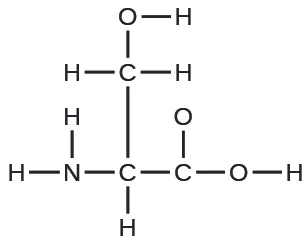(b) urea: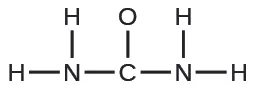(c) pyruvic acid: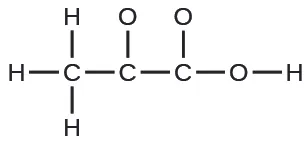(d) uracil: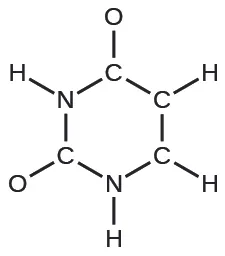(e) carbonic acid: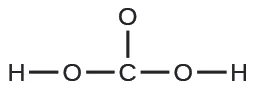40.

A compound with a molar mass of about 28 g/mol contains 85.7% carbon and 14.3% hydrogen by mass. Write the Lewis structure for a molecule of the compound.

41.

A compound with a molar mass of about 42 g/mol contains 85.7% carbon and 14.3% hydrogen by mass. Write the Lewis structure for a molecule of the compound.

42.

Two arrangements of atoms are possible for a compound with a molar mass of about 45 g/mol that contains 52.2% C, 13.1% H, and 34.7% O by mass. Write the Lewis structures for the two molecules.

43.

How are single, double, and triple bonds similar? How do they differ?

### 7.4Formal Charges and Resonance

44.

Write resonance forms that describe the distribution of electrons in each of these molecules or ions.

(a) selenium dioxide, OSeO

(b) nitrate ion, $NO3−NO3−$

(c) nitric acid, HNO3 (N is bonded to an OH group and two O atoms)

(d) benzene, C6H6: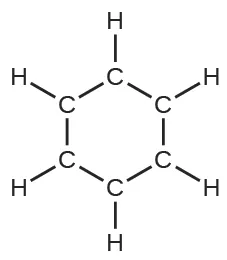(e) the formate ion: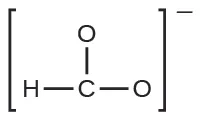45.

Write resonance forms that describe the distribution of electrons in each of these molecules or ions.

(a) sulfur dioxide, SO2

(b) carbonate ion, $CO32−CO32−$

(c) hydrogen carbonate ion, $HCO3−HCO3−$ (C is bonded to an OH group and two O atoms)

(d) pyridine: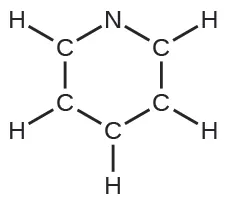(e) the allyl ion: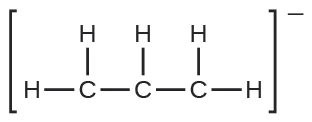46.

Write the resonance forms of ozone, O3, the component of the upper atmosphere that protects the Earth from ultraviolet radiation.

47.

Sodium nitrite, which has been used to preserve bacon and other meats, is an ionic compound. Write the resonance forms of the nitrite ion, $NO2–.NO2–.$

48.

In terms of the bonds present, explain why acetic acid, CH3CO2H, contains two distinct types of carbon-oxygen bonds, whereas the acetate ion, formed by loss of a hydrogen ion from acetic acid, only contains one type of carbon-oxygen bond. The skeleton structures of these species are shown: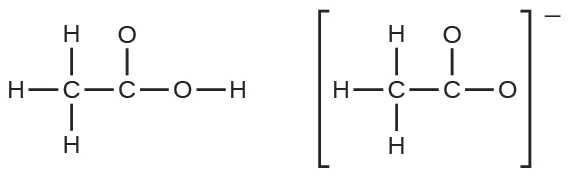49.

Write the Lewis structures for the following, and include resonance structures where appropriate. Indicate which has the strongest carbon-oxygen bond.

(a) CO2

(b) CO

50.

Toothpastes containing sodium hydrogen carbonate (sodium bicarbonate) and hydrogen peroxide are widely used. Write Lewis structures for the hydrogen carbonate ion and hydrogen peroxide molecule, with resonance forms where appropriate.

51.

Determine the formal charge of each element in the following:

(a) HCl

(b) CF4

(c) PCl3

(d) PF5

52.

Determine the formal charge of each element in the following:

(a) H3O+

(b) $SO42−SO42−$

(c) NH3

(d) $O22−O22−$

(e) H2O2

53.

Calculate the formal charge of chlorine in the molecules Cl2, BeCl2, and ClF5.

54.

Calculate the formal charge of each element in the following compounds and ions:

(a) F2CO

(b) NO

(c) $BF4−BF4−$

(d) $SnCl3−SnCl3−$

(e) H2CCH2

(f) ClF3

(g) SeF6

(h) $PO43−PO43−$

55.

Draw all possible resonance structures for each of these compounds. Determine the formal charge on each atom in each of the resonance structures:

(a) O3

(b) SO2

(c) $NO2−NO2−$

(d) $NO3−NO3−$

56.

Based on formal charge considerations, which of the following would likely be the correct arrangement of atoms in nitrosyl chloride: ClNO or ClON?

57.

Based on formal charge considerations, which of the following would likely be the correct arrangement of atoms in hypochlorous acid: HOCl or OClH?

58.

Based on formal charge considerations, which of the following would likely be the correct arrangement of atoms in sulfur dioxide: OSO or SOO?

59.

Draw the structure of hydroxylamine, H3NO, and assign formal charges; look up the structure. Is the actual structure consistent with the formal charges?

60.

Iodine forms a series of fluorides (listed here). Write Lewis structures for each of the four compounds and determine the formal charge of the iodine atom in each molecule:

(a) IF

(b) IF3

(c) IF5

(d) IF7

61.

Write the Lewis structure and chemical formula of the compound with a molar mass of about 70 g/mol that contains 19.7% nitrogen and 80.3% fluorine by mass, and determine the formal charge of the atoms in this compound.

62.

Which of the following structures would we expect for nitrous acid? Determine the formal charges: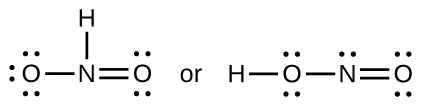63.

Sulfuric acid is the industrial chemical produced in greatest quantity worldwide. About 90 billion pounds are produced each year in the United States alone. Write the Lewis structure for sulfuric acid, H2SO4, which has two oxygen atoms and two OH groups bonded to the sulfur.

### 7.5Strengths of Ionic and Covalent Bonds

64.

Which bond in each of the following pairs of bonds is the strongest?

(a) C–C or $C=CC=C$

(b) C–N or $C≡NC≡N$

(c)$C≡OC≡O$ or $C=OC=O$

(d) H–F or H–Cl

(e) C–H or O–H

(f) C–N or C–O

65.

Using the bond energies in Table 7.2, determine the approximate enthalpy change for each of the following reactions:

(a) $H2(g)+Br2(g)⟶2HBr(g)H2(g)+Br2(g)⟶2HBr(g)$

(b) $CH4(g)+I2(g)⟶CH3I(g)+HI(g)CH4(g)+I2(g)⟶CH3I(g)+HI(g)$

(c) $C2H4(g)+3O2(g)⟶2CO2(g)+2H2O(g)C2H4(g)+3O2(g)⟶2CO2(g)+2H2O(g)$

66.

Using the bond energies in Table 7.2, determine the approximate enthalpy change for each of the following reactions:

(a) $Cl2(g)+3F2(g)⟶2ClF3(g)Cl2(g)+3F2(g)⟶2ClF3(g)$

(b) $H2C=CH2(g)+H2(g)⟶H3CCH3(g)H2C=CH2(g)+H2(g)⟶H3CCH3(g)$

(c) $2C2H6(g)+7O2(g)⟶4CO2(g)+6H2O(g)2C2H6(g)+7O2(g)⟶4CO2(g)+6H2O(g)$

67.

When a molecule can form two different structures, the structure with the stronger bonds is usually the more stable form. Use bond energies to predict the correct structure of the hydroxylamine molecule: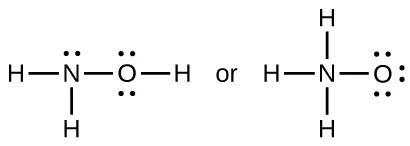68.

How does the bond energy of HCl(g) differ from the standard enthalpy of formation of HCl(g)?

69.

Using the standard enthalpy of formation data in Appendix G, show how the standard enthalpy of formation of HCl(g) can be used to determine the bond energy.

70.

Using the standard enthalpy of formation data in Appendix G, calculate the bond energy of the carbon-sulfur double bond in CS2.

71.

Using the standard enthalpy of formation data in Appendix G, determine which bond is stronger: the S–F bond in SF4(g) or in SF6(g)?

72.

Using the standard enthalpy of formation data in Appendix G, determine which bond is stronger: the P–Cl bond in PCl3(g) or in PCl5(g)?

73.

Complete the following Lewis structure by adding bonds (not atoms), and then indicate the longest bond: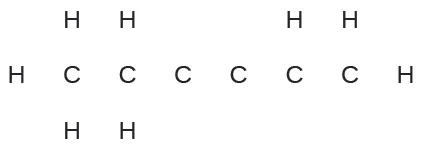74.

Use the bond energy to calculate an approximate value of ΔH for the following reaction. Which is the more stable form of FNO2?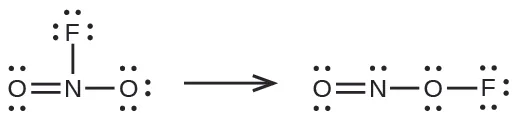75.

Use principles of atomic structure to answer each of the following:1

(a) The radius of the Ca atom is 197 pm; the radius of the Ca2+ ion is 99 pm. Account for the difference.

(b) The lattice energy of CaO(s) is –3460 kJ/mol; the lattice energy of K2O is –2240 kJ/mol. Account for the difference.

(c) Given these ionization values, explain the difference between Ca and K with regard to their first and second ionization energies.

Element First Ionization Energy (kJ/mol) Second Ionization Energy (kJ/mol)
K 419 3050
Ca 590 1140

(d) The first ionization energy of Mg is 738 kJ/mol and that of Al is 578 kJ/mol. Account for this difference.

76.

The lattice energy of LiF is 1023 kJ/mol, and the Li–F distance is 200.8 pm. NaF crystallizes in the same structure as LiF but with a Na–F distance of 231 pm. Which of the following values most closely approximates the lattice energy of NaF: 510, 890, 1023, 1175, or 4090 kJ/mol? Explain your choice.

77.

For which of the following substances is the least energy required to convert one mole of the solid into separate ions?

(a) MgO

(b) SrO

(c) KF

(d) CsF

(e) MgF2

78.

The reaction of a metal, M, with a halogen, X2, proceeds by an exothermic reaction as indicated by this equation: $M(s)+X2(g)⟶MX2(s).M(s)+X2(g)⟶MX2(s).$ For each of the following, indicate which option will make the reaction more exothermic. Explain your answers.

(b) a high ionization energy vs. a low ionization energy for M

(c) an increasing bond energy for the halogen

(d) a decreasing electron affinity for the halogen

(e) an increasing size of the anion formed by the halogen

79.

The lattice energy of LiF is 1023 kJ/mol, and the Li–F distance is 201 pm. MgO crystallizes in the same structure as LiF but with a Mg–O distance of 205 pm. Which of the following values most closely approximates the lattice energy of MgO: 256 kJ/mol, 512 kJ/mol, 1023 kJ/mol, 2046 kJ/mol, or 4008 kJ/mol? Explain your choice.

80.

Which compound in each of the following pairs has the larger lattice energy? Note: Mg2+ and Li+ have similar radii; O2– and F have similar radii. Explain your choices.

(a) MgO or MgSe

(b) LiF or MgO

(c) Li2O or LiCl

(d) Li2Se or MgO

81.

Which compound in each of the following pairs has the larger lattice energy? Note: Ba2+ and

(a) K2O or Na2O

(b) K2S or BaS

(c) KCl or BaS

(d) BaS or BaCl2

82.

Which of the following compounds requires the most energy to convert one mole of the solid into separate ions?

(a) MgO

(b) SrO

(c) KF

(d) CsF

(e) MgF2

83.

Which of the following compounds requires the most energy to convert one mole of the solid into separate ions?

(a) K2S

(b) K2O

(c) CaS

(d) Cs2S

(e) CaO

84.

The lattice energy of KF is 794 kJ/mol, and the interionic distance is 269 pm. The Na–F

distance in NaF, which has the same structure as KF, is 231 pm. Which of the following values is the closest approximation of the lattice energy of NaF: 682 kJ/mol, 794 kJ/mol, 924 kJ/mol, 1588 kJ/mol, or 3175 kJ/mol? Explain your answer.

### 7.6Molecular Structure and Polarity

85.

Explain why the HOH molecule is bent, whereas the HBeH molecule is linear.

86.

What feature of a Lewis structure can be used to tell if a molecule’s (or ion’s) electron-pair geometry and molecular structure will be identical?

87.

Explain the difference between electron-pair geometry and molecular structure.

88.

Why is the H–N–H angle in NH3 smaller than the H–C–H bond angle in CH4? Why is the H–N–H angle in $NH4+NH4+$ identical to the H–C–H bond angle in CH4?

89.

Explain how a molecule that contains polar bonds can be nonpolar.

90.

As a general rule, MXn molecules (where M represents a central atom and X represents terminal atoms; n = 2 – 5) are polar if there is one or more lone pairs of electrons on M. NH3 (M = N, X = H, n = 3) is an example. There are two molecular structures with lone pairs that are exceptions to this rule. What are they?

91.

Predict the electron pair geometry and the molecular structure of each of the following molecules or ions:

(a) SF6

(b) PCl5

(c) BeH2

(d) $CH3+CH3+$

92.

Identify the electron pair geometry and the molecular structure of each of the following molecules or ions:

(a) $IF6+IF6+$

(b) CF4

(c) BF3

(d) $SiF5−SiF5−$

(e) BeCl2

93.

What are the electron-pair geometry and the molecular structure of each of the following molecules or ions?

(a) ClF5

(b) $ClO2−ClO2−$

(c) $TeCl42−TeCl42−$

(d) PCl3

(e) SeF4

(f) $PH2−PH2−$

94.

Predict the electron pair geometry and the molecular structure of each of the following ions:

(a) H3O+

(b) $PCl4−PCl4−$

(c) $SnCl3+SnCl3+$

(d) $BrCl4−BrCl4−$

(e) ICl3

(f) XeF4

(g) SF2

95.

Identify the electron pair geometry and the molecular structure of each of the following molecules:

(a) ClNO (N is the central atom)

(b) CS2

(c) Cl2CO (C is the central atom)

(d) Cl2SO (S is the central atom)

(e) SO2F2 (S is the central atom)

(f) XeO2F2 (Xe is the central atom)

(g) $ClOF2+ClOF2+$ (Cl is the central atom)

96.

Predict the electron pair geometry and the molecular structure of each of the following:

(a) IOF5 (I is the central atom)

(b) POCl3 (P is the central atom)

(c) Cl2SeO (Se is the central atom)

(d) ClSO+ (S is the central atom)

(e) F2SO (S is the central atom)

(f) $NO2−NO2−$

(g) $SiO44−SiO44−$

97.

Which of the following molecules and ions contain polar bonds? Which of these molecules and ions have dipole moments?

(a) ClF5

(b) $ClO2−ClO2−$

(c) $TeCl42−TeCl42−$

(d) PCl3

(e) SeF4

(f) $PH2−PH2−$

(g) XeF2

98.

Which of these molecules and ions contain polar bonds? Which of these molecules and ions have dipole moments?

(a) H3O+

(b) $PCl4−PCl4−$

(c) $SnCl3−SnCl3−$

(d) $BrCl4−BrCl4−$

(e) ICl3

(f) XeF4

(g) SF2

99.

Which of the following molecules have dipole moments?

(a) CS2

(b) SeS2

(c) CCl2F2

(d) PCl3 (P is the central atom)

(e) ClNO (N is the central atom)

100.

Identify the molecules with a dipole moment:

(a) SF4

(b) CF4

(c) Cl2CCBr2

(d) CH3Cl

(e) H2CO

101.

The molecule XF3 has a dipole moment. Is X boron or phosphorus?

102.

The molecule XCl2 has a dipole moment. Is X beryllium or sulfur?

103.

Is the Cl2BBCl2 molecule polar or nonpolar?

104.

There are three possible structures for PCl2F3 with phosphorus as the central atom. Draw them and discuss how measurements of dipole moments could help distinguish among them.

105.

Describe the molecular structure around the indicated atom or atoms:

(a) the sulfur atom in sulfuric acid, H2SO4 [(HO)2SO2]

(b) the chlorine atom in chloric acid, HClO3 [HOClO2]

(c) the oxygen atom in hydrogen peroxide, HOOH

(d) the nitrogen atom in nitric acid, HNO3 [HONO2]

(e) the oxygen atom in the OH group in nitric acid, HNO3 [HONO2]

(f) the central oxygen atom in the ozone molecule, O3

(g) each of the carbon atoms in propyne, CH3CCH

(h) the carbon atom in Freon, CCl2F2

(i) each of the carbon atoms in allene, H2CCCH2

106.

Draw the Lewis structures and predict the shape of each compound or ion:

(a) CO2

(b) $NO2−NO2−$

(c) SO3

(d) $SO32−SO32−$

107.

A molecule with the formula AB2, in which A and B represent different atoms, could have one of three different shapes. Sketch and name the three different shapes that this molecule might have. Give an example of a molecule or ion for each shape.

108.

A molecule with the formula AB3, in which A and B represent different atoms, could have one of three different shapes. Sketch and name the three different shapes that this molecule might have. Give an example of a molecule or ion that has each shape.

109.

Draw the Lewis electron dot structures for these molecules, including resonance structures where appropriate:

(a) $CS32−CS32−$

(b) CS2

(c) CS

(d) predict the molecular shapes for $CS32−CS32−$ and CS2 and explain how you arrived at your predictions

110.

What is the molecular structure of the stable form of FNO2? (N is the central atom.)

111.

A compound with a molar mass of about 42 g/mol contains 85.7% carbon and 14.3% hydrogen. What is its molecular structure?

112.

Use the simulation to perform the following exercises for a two-atom molecule:

(a) Adjust the electronegativity value so the bond dipole is pointing toward B. Then determine what the electronegativity values must be to switch the dipole so that it points toward A.

(b) With a partial positive charge on A, turn on the electric field and describe what happens.

(c) With a small partial negative charge on A, turn on the electric field and describe what happens.

(d) Reset all, and then with a large partial negative charge on A, turn on the electric field and describe what happens.

113.

Use the simulation to perform the following exercises for a real molecule. You may need to rotate the molecules in three dimensions to see certain dipoles.

(a) Sketch the bond dipoles and molecular dipole (if any) for O3. Explain your observations.

(b) Look at the bond dipoles for NH3. Use these dipoles to predict whether N or H is more electronegative.

(c) Predict whether there should be a molecular dipole for NH3 and, if so, in which direction it will point. Check the molecular dipole box to test your hypothesis.

114.

Use the Molecule Shape simulator to build a molecule. Starting with the central atom, click on the double bond to add one double bond. Then add one single bond and one lone pair. Rotate the molecule to observe the complete geometry. Name the electron group geometry and molecular structure and predict the bond angle. Then click the check boxes at the bottom and right of the simulator to check your answers.

115.

Use the Molecule Shape simulator to explore real molecules. On the Real Molecules tab, select H2O. Switch between the “real” and “model” modes. Explain the difference observed.

116.

Use the Molecule Shape simulator to explore real molecules. On the Real Molecules tab, select “model” mode and S2O. What is the model bond angle? Explain whether the “real” bond angle should be larger or smaller than the ideal model angle.

### Footnotes

• 1This question is taken from the Chemistry Advanced Placement Examination and is used with the permission of the Educational Testing Service.
Order a print copy

As an Amazon Associate we earn from qualifying purchases.